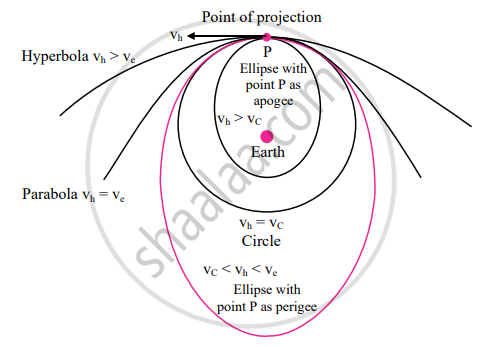# Answer the following question in detail. Draw a well labelled diagram to show different trajectories depending upon the tangential projection speed. - Physics

Diagram

Answer the following question in detail.

Draw a well labelled diagram to show different trajectories depending upon the tangential projection speed.

#### Solutionvh = horizontal speed of projection
vc = critical velocity
ve = escape velocity

Concept: Earth Satellites
Is there an error in this question or solution?

#### APPEARS IN

Balbharati Physics 11th Standard Maharashtra State Board
Chapter 5 Gravitation
Exercises | Q 3. (iv) | Page 98